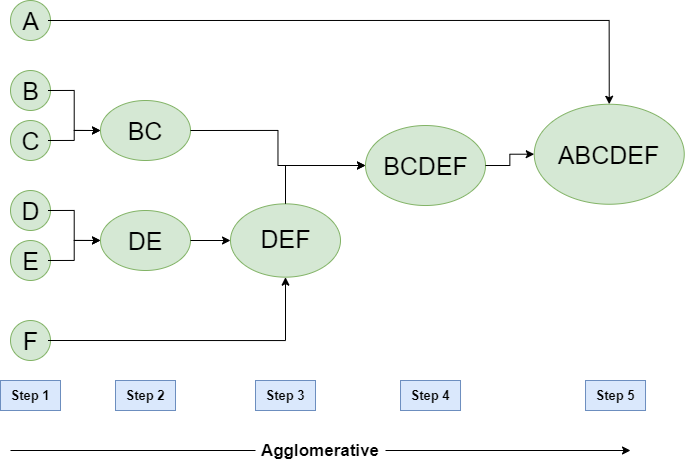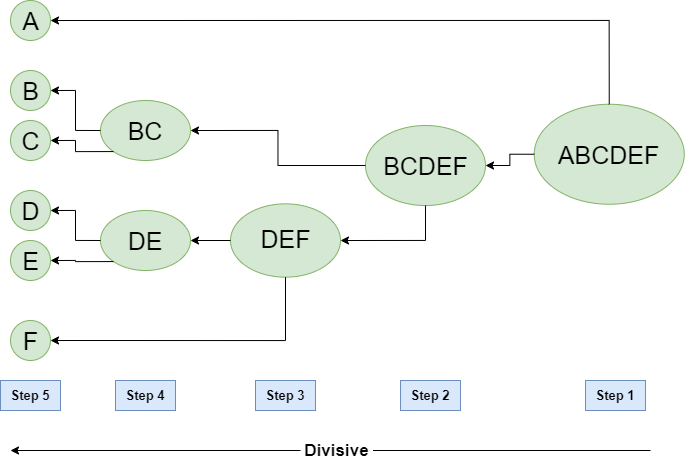Related Articles

# Hierarchical Clustering in Data Mining

• Difficulty Level : Easy
• Last Updated : 05 Feb, 2020

A Hierarchical clustering method works via grouping data into a tree of clusters. Hierarchical clustering begins by treating every data points as a separate cluster. Then, it repeatedly executes the subsequent steps:

1. Identify the 2 clusters which can be closest together, and
2. Merge the 2 maximum comparable clusters. We need to continue these steps until all the clusters are merged together.

In Hierarchical Clustering, the aim is to produce a hierarchical series of nested clusters. A diagram called Dendrogram (A Dendrogram is a tree-like diagram that statistics the sequences of merges or splits) graphically represents this hierarchy and is an inverted tree that describes the order in which factors are merged (bottom-up view) or cluster are break up (top-down view).

The basic method to generate hierarchical clustering are:

1. Agglomerative:
Initially consider every data point as an individual Cluster and at every step, merge the nearest pairs of the cluster. (It is a bottom-up method). At first everydata set set is considered as individual entity or cluster. At every iteration, the clusters merge with different clusters until one cluster is formed.

Algorithm for Agglomerative Hierarchical Clustering is:

• Calculate the similarity of one cluster with all the other clusters (calculate proximity matrix)
• Consider every data point as a individual cluster
• Merge the clusters which are highly similar or close to each other.
• Recalculate the proximity matrix for each cluster
• Repeat Step 3 and 4 until only a single cluster remains.

Let’s see the graphical representation of this algorithm using a dendrogram.

Note:
This is just a demonstration of how the actual algorithm works no calculation has been performed below all the proximity among the clusters are assumed.

Let’s say we have six data points A, B, C, D, E, F.Figure – Agglomerative Hierarchical clustering
• Step-1:
Consider each alphabet as a single cluster and calculate the distance of one cluster from all the other clusters.
• Step-2:
In the second step comparable clusters are merged together to form a single cluster. Let’s say cluster (B) and cluster (C) are very similar to each other therefore we merge them in the second step similarly with cluster (D) and (E) and at last, we get the clusters
[(A), (BC), (DE), (F)]
• Step-3:
We recalculate the proximity according to the algorithm and merge the two nearest clusters([(DE), (F)]) together to form new clusters as [(A), (BC), (DEF)]
• Step-4:
Repeating the same process; The clusters DEF and BC are comparable and merged together to form a new cluster. We’re now left with clusters [(A), (BCDEF)].
• Step-5:
At last the two remaining clusters are merged together to form a single cluster [(ABCDEF)].

2. Divisive:
We can say that the Divisive Hierarchical clustering is precisely the opposite of the Agglomerative Hierarchical clustering. In Divisive Hierarchical clustering, we take into account all of the data points as a single cluster and in every iteration, we separate the data points from the clusters which aren’t comparable. In the end, we are left with N clusters.Figure – Divisive Hierarchical clustering

Attention reader! Don’t stop learning now. Get hold of all the important CS Theory concepts for SDE interviews with the CS Theory Course at a student-friendly price and become industry ready.

My Personal Notes arrow_drop_up